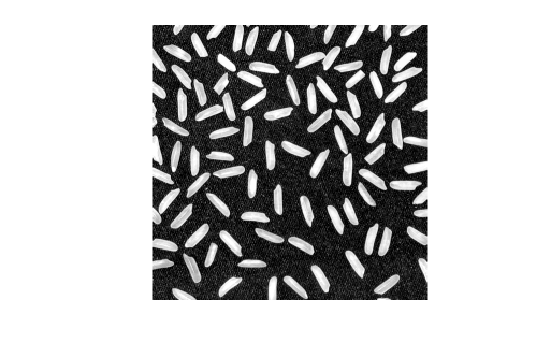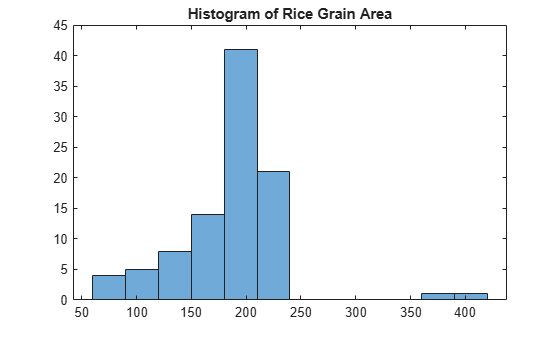Documentation

Correct Nonuniform Illumination and Analyze Foreground Objects

This example shows how to enhance an image as a preprocessing step before analysis. In this example, you correct the nonuniform background illumination and convert the image into a binary image to make it easy to identify foreground objects (individual grains of rice). You can then analyze the objects, such as finding the area of each grain of rice, and you can compute statistics for all objects in the image.

Preprocess the Image

Read an image into the workspace.

imshow(I)The background illumination is brighter in the center of the image than at the bottom. Preprocess the image to make the background illumination more uniform.

As a first step, remove all the foreground (rice grains) using morphological opening. The opening operation removes small objects that cannot completely contain the structuring element. Define a disk-shaped structuring element with a radius of 15, which does fit entirely inside a single grain of rice.

se = strel('disk',15)
se =
strel is a disk shaped structuring element with properties:

Neighborhood: [29x29 logical]
Dimensionality: 2

To perform the morphological opening, use imopen with the structuring element.

background = imopen(I,se);
imshow(background)Subtract the background approximation image, background, from the original image, I, and view the resulting image. After subtracting the adjusted background image from the original image, the resulting image has a uniform background but is now a bit dark for analysis.

I2 = I - background;
imshow(I2)Use imadjust to increase the contrast of the processed image I2 by saturating 1% of the data at both low and high intensities and by stretching the intensity values to fill the uint8 dynamic range.

imshow(I3)Note that the prior two steps could be replaced by a single step using imtophat which first calculates the morphological opening and then subtracts it from the original image.

I2 = imtophat(I,strel('disk',15));

Create a binary version of the processed image so you can use toolbox functions for analysis. Use the imbinarize function to convert the grayscale image into a binary image. Remove background noise from the image with the bwareaopen function.

bw = imbinarize(I3);
bw = bwareaopen(bw,50);
imshow(bw)Identify Objects in the Image

Now that you have created a binary version of the original image you can perform analysis of objects in the image.

Find all the connected components (objects) in the binary image. The accuracy of your results depends on the size of the objects, the connectivity parameter (4, 8, or arbitrary), and whether or not any objects are touching (in which case they could be labeled as one object). Some of the rice grains in the binary image bw are touching.

cc = bwconncomp(bw,4)
cc = struct with fields:
Connectivity: 4
ImageSize: [256 256]
NumObjects: 95
PixelIdxList: {1x95 cell}

cc.NumObjects
ans = 95

View the rice grain that is labeled 50 in the image.

grain = false(size(bw));
grain(cc.PixelIdxList{50}) = true;
imshow(grain)Visualize all the connected components in the image by creating a label matrix and then displaying it as a pseudocolor indexed image.

Use labelmatrix to create a label matrix from the output of bwconncomp. Note that labelmatrix stores the label matrix in the smallest numeric class necessary for the number of objects.

labeled = labelmatrix(cc);
whos labeled
Name           Size             Bytes  Class    Attributes

labeled      256x256            65536  uint8

Use label2rgb to choose the colormap, the background color, and how objects in the label matrix map to colors in the colormap. In the pseudocolor image, the label identifying each object in the label matrix maps to a different color in an associated colormap matrix.

RGB_label = label2rgb(labeled,'spring','c','shuffle');
imshow(RGB_label)Compute Area-Based Statistics

Compute the area of each object in the image using regionprops. Each rice grain is one connected component in the cc structure.

graindata = regionprops(cc,'basic')
graindata=95×3 struct
Area
Centroid
BoundingBox

Create a new vector grain_areas, which holds the area measurement for each grain.

grain_areas = [graindata.Area];

Find the area of the 50th component.

grain_areas(50)
ans = 194

Find and display the grain with the smallest area.

[min_area, idx] = min(grain_areas)
min_area = 61
idx = 16
grain = false(size(bw));
grain(cc.PixelIdxList{idx}) = true;
imshow(grain)Use the histogram command to create a histogram of rice grain areas.

histogram(grain_areas)
title('Histogram of Rice Grain Area')